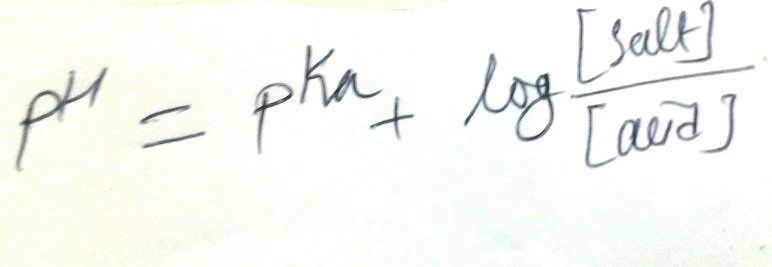# How to calculate pKa of phosphate buffer?

(Last Updated On: October 14, 2022)Henderson Hasselbalch equation can be employed to calculate the concentration of individual components of a buffer system.

The pKa of phosphate buffer can be calculated by using a mathematical expression. Phosphate buffer is an important buffer system used in biochemistry laboratories. Phosphate buffer is essential for experiments involving proteins and enzymes. It maintains the pH of the solution and also prevents a drastic change in the pH. Therefore, we have to be familiar with the preparation of the phosphate buffer and how it works.

## Calculation of pKa of phosphate buffer

Since phosphate buffer consists of two components; dihydrogen phosphate (H2PO4) and hydrogen phosphate (HPO4-2), there is a dissociation constant that we call the acidity constant of phosphate buffer. Phosphoric acid is a tri protic acid and, therefore, there are three acidity constants for this acid. However, our concern for the pKa of phosphate buffer is with the acidity constant of dihydrogen phosphate (H2PO4). Acidity constants of phosphoric acid are, H3PO4 (), H2PO4 (),  and HPO4-2 ()

Phosphate buffer involves in the ionization of H2PO4 to HPO4-2 and vice versa. Therefore, we will use the acidity constant K2 to determine the pKa value.  Since pK1 is a negative logarithm of the acidity constant, pKa will be log(K2) or log(6.2*10-8) or 7.21. To know more about the dissociation constants of weak acids and bases, please visit the Chemistry Libretexts website.

Therefore, the pKa value of the phosphate buffer is 7.21. We can now use it to prepare the phosphate buffer of pH 7.21 ± 1 with a definite ionic strength. Knowing the pKa of a buffer is very important during the buffer solution preparation.

## Preparation of the phosphate buffer

Using the Henderson-Hasselbalch equation, you can calculate the required concentration of each component of the phosphate buffer of the desired pH. You just have to put the pKa that you determined above (7.21) and the desired pH of the solution (that can be 7.21 ± 1) and you will be able to determine the concentration of H2PO4- and HPO4-2. It’s just easy to calculate the concentration of individual components H2PO4- and HPO4-2 using the mathematical expression Henderson-Hasselbalch equation as given below. For phosphate buffer, H2PO4- is the acid, and HPO4-2. is the salt.

Using the Henderson-Hasselbalch equation; you can calculate the required components of the phosphate buffer or if you want you can also use an online phosphate buffer calculator. I have recently developed an online calculator only for you so that you can easily determine the necessary amounts of individual components of the phosphate buffer without any calculations.